## GIS - Computational Problems: § 4: Advanced Problems

#### Instructors: G.X. Ritter and M.S.Schmalz

The majority of GIS datasets are currently represented in vector format, and have an inherent representational error that arises from sensor errors as well as from discretization or polygonalization processes that we discussed in detail in Section 2. GIS algorithms propagate this dataset error through various stages of computation, yielding a GIS product or result that often has unexpected errors. Such errors influence coregistration (e.g., map overlay) and tend to corrupt the derivation of range or elevation data from stereo imagery. This further impacts the integration of surface models (e.g., spline models based on elevation data) with GIS datasets.

Section Overview. In this section, we discuss errors in GIS datasets as well as error and complexity measures, then investigate the effect of such errors on elevation and surface modelling with GIS. The section is structured as follows:

4.1. Estimating Error in GIS Datasets
4.2. Prediction of Error and Complexity in GIS Algorithms
4.3. Determining Elevation from Stereo Images in GIS Data
4.4. Integrating Surface Models with Elevation Data

In Section 4.1, we present an in-depth discussion of errors in spatial datasets, together with measures for quantifying such errors and various types of error analysis. Section 4.2 details techniques for error analysis as well as the estimation of complexity in GIS algorithms, both of which are required for the design and implementation of accurate, efficient software. The difficult problem of automatic analysis of stereophotogrammetric data is presented in Section 4.3, and an extension of this problem to multi-modal GIS (e.g., derived and measured elevation data) is discussed in Section 4.4.

Goals. For the purposes of the current course, goals for this section include:

1. Learning error/complexity analysis terminology and background;
2. Exploring two error-related problems instantiated as stereophotogrammetry and data integration of vector- and image-format elevation data;
3. Understanding the underlying issues of error detection, measurement, modelling, and management in GIS; and
4. Prediction of computational cost and accuracy (e.g., the space-time-error bandwidth product) for a given GIS algorithm.

We begin our discussion with a distinction between cartographic error and GIS error.

### 4.1. Estimating Error in GIS Datasets

Note that map accuracy is a relatively minor issue in cartography. Users of maps are rarely aware of this problem, due to their familiarity with map notation and its underlying assumptions. For example, if a map shows that a drainage ditch runs parallel to a road, one assumes from world knowledge that the ditch is located close to the road. However, on the map, for purposes of cartographic license, the map may appear to be offset laterally from the road by as much as the width of the road.

#### 4.1.1. Background.

In contrast to cartographic practice, GIS has evolved under the following circumstances:

1. GIS precision is limited only by computational hardware, for example, ALU register size, cost of I/O and memory, or speed of storage devices.

2. All spatial data have limited accuracy which may be expressed in terms of positional error, abstraction or generalization error, measurement error, etc. Consider the following examples:

• Surveying error: Systematic or random errors in measurement of distance or angle.

• Decision error: Vague geographic relations such as west-of.

• Classification error: Soil type tends to occur as a gradual transition in Nature but is characterized by sharp between-class boundaries in GIS (e.g., sand, loam, or silt).

• Stationarity error: Population density, land use information, or income data not uniform over a given spatial area over which the data is averaged.

• Relational error: Position and attribute error may not be analytically related. For example, terrain elevation is not necessarily a function of lateral position in monocular satellite imagery, but is related to position in binocular (stereoscopic) imaging.

3. Precision of GIS processing exceeds data accuracy -- GIS processing is performed at high (16- to 32-bit) arithmetic precision, but GIS data have much lower precision (e.g., four to ten parts per 10,000).

4. In conventional map analysis, precision is usually adapted to accuracy

• Precision limit of paper is approximately 0.5mm or one linewidth, and map error increases with humidity and use, due to paper warpage.

• Area accuracy is thus approximately m2.

• Map processes (planimetry, dot counting, transparency overlay) have approximately the same error (one linewidth).

5. The ability to change scale and combine data at different scales implies that GIS precision is not necessarily adapted to accuracy.

• Example. GIS systems generally do not warn users if datasets of different scale (e.g., 1:24,000 vs. 1:1,000,000) are combined and the result is displayed at 1:50,000 scale. This causes loss (aliasing) of information from the high- (low-) resolution dataset.

• Observation. Most vector-based systems perform vector operations (line intersection, overlay, area computation) at full computational precision, without regard for dataset accuracy. Users are often surprised by errors when GIS is validated against ground truth.

6. The accuracy of complex spatial objects is not well understood. The accuracy of points, lines, and simple neighborhood areas (e.g., circle or rectangle) has been analyzed extensively. However, methods of abstracting error as a function of lateral position is not clear.

7. The goal of GIS error analysis is a measure of uncertainty associated with every GIS product (e.g., datasets, maps, and analyses).

• Ideal: A set of confidence limits with each map.

• Problems: Cartographic license, map warpage and digitization errors, other errors listed previously can produce a diversity of error modes and magnitudes in GIS datasets.

• Reality: Error measures are likely on points and lines, maybe some for area, since supporting theory can be derived rigorously from map coordinate information.

Hence, the key issues in this section are measurement, estimation, and prediction of GIS error. The following observations pertain:

• GIS attributes are often non-numeric and cannot (in some cases) be indexed in a physically faithful way by a subset of the real numbers.

• The root problem in this case can be stated as follows:

• All physical science is defined in terms of mathematics, which is based on measurement theory and the formal logic.

• In contrast, language and humanistic pursuits (from which the process of classification arises) have as yet no rigorous supporting or descriptive mathematics.

• Thus, attributes or labels, which are linguistic entities, cannot in many instances be rigorously assigned numerical values or ranked in a physically significant way.

#### 4.1.2. Current Approaches to GIS Error Modelling.

The following discussion is adapted from Veregin (1994).

The error modelling process can be decomposed into the following steps or levels:

Level 1. Error source and mode isolation -- Determine at what locations in a given algorithm errors arise, and in what form such errors occur.

Level 2. Error detection and measurement -- Devise and implement test procedures for detecting and estimating error deterministically or stochastically.

Level 3. Error propagation modelling -- Predict or estimate the accrual of error as a computational cascade comprised of a sequence of operations.

Level 4. Strategies for error management -- Determine methods for carrying out GIS computations to achieve minimum output error.

Level 5. Strategies for error "reduction" -- Note that the common assumption is erroneous, namely, that error can be reduced via summation over error distributions. In practice, such distributions are often asymmetrical with nonzero means. Indeed, the assumption that error can be nontrivially reduced derives from convenient pedagogic examples that employ symmetric distributions with zero mean or assume infinite computational precision. In practice, "error reduction" often strives to re-order the processes of a GIS algorithm, or substitute less erroneous processes, which is a type of error management.

At Level 1 (error isolation), the following types of error are found:

• Positional, Locational, or Cartographic error, which derive from map coordinate data;

• Thematic, Atrribute, or Descriptive error, pertaining directly to GIS attributes;

• Measurement error, describing imprecision in cartographic or thematic features;

• Conceptual error, which occurs when translating field data into map objects (similar to Generalization error);

• Quantitative error respective to interval and ratio data (on R); and

• Qualitative error respective to nominal or ordinal data (indexed by N).

In this course, we will concentrate upon positional and attribute error primarily, and thus examine Levels 1-5 of the preceding hierarchy for error sources.

Level 1 (Error isolation) sources of error are classified by type as shown in the following examples:

Positional: GPS and theodolite (surveying equipment) error, or object location errors due to misunderstanding of cartographic license.

Attribute: Poor assessment of attributes to due measurement, conceptual, quantitative or qualitative error, as well as systematic (e.g., operator) error in manual classification systems.

Level 2 (Error detection and measurement) focuses on methods of assessing accuracy levels in spatial data, for example:

• Digitization practices can be scrutinized to detect systematic error or imprecision (e.g., a loose or misaligned piece of mechanical equipment). Such problems can lead to positional, measurement, and conceptual error.

• Similarly, spaceborne or airborne imaging platforms can exhibit positional error due to vibration, measurement error due to spectral filter response drift with time and temperature, and conceptual error due to mis-classification of terrain features by spectral signatures that are specified erroneously a priori.

Level 3 (Error propagation modelling) focuses on error propagation and error production, which are distinguished in the following examples:

• Error Propagation always increases the error magnitude at the output of an operation with respect to the error magnitude at its input. Theory for estimating error propagation is well known for linear systems, but is less well established for nonlinear systems.

• Error Production (computational error) results primarily from roundoff errors in arithmetic operations and truncation of series approximations. An additional source of computational error is the approximation of transcendental functions by lookup tables.

Level 4 (Error management) focuses on coping with error and making specifiably accurate decisions in the presence of error. In GIS, this can involve:

• Specification of minimum accuracy standards for GIS product acceptance, such as:

• Thematic- or attribute-specific standards
• Accuracy levels for cartographic feature specification

• Methods of inference derived from artificial intelligence and information theory, especially concerning decision in the presence of uncertainty.

Level 5 (Strategies for error reduction) includes but is not limited to the following methods:

• Heuristics and decision trees for minimizing or reducing error propagation, for example, placing more erroneous operations at the end of a computational cascade, which can involve out-of-order execution of instructions;

• Use of expert knowledge, replacement data, or checking procedures, as follows:

• Expert Knowledge: For example, which input (e.g., a given imaging sensor or spectral band of a multispectral camera) to use when detecting a given object type, with the goal of achieving high signal-to-noise ratio (SNR).

• Replacement Data: Use a lower-noise dataset for a given high-error computation (although there may be tradeoffs due to noise and resolution)

• Checking Procedures: Use fast, low-precision operations until error accumulates to a prespecified (estimated) level, then switch to slower, more precise operations (e.g., using more terms in a series approximation of a transcendental function).

#### 4.1.3. Examples of Key GIS Errors.

The majority of GIS errors can be exemplified by the following three case studies. In Section 4.1.3.1, we discuss polygon fragmentation in map overlay. Section 4.1.3.2 contains an overview of thematic and attribute errors, and Section 4.1.3.3 summarizes category-based error.

#### 4.1.3.1. Case Study: Polygon Fragmentation Error.

Concept. Let there exist multiple maps ai, where i = 1..n, which are defined on a two-dimensional domain X. In practice, due to misalignments between map features in each map, spurious polygons result from superimposing the polygon boundaries of each ai.

Theory. Given aiFX, where 1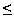in, let there be ki polygons per layer. According to McAlpine and Cook [McA71], an estimate of the number of spurious polygons is given by:

kovl = (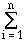ki1/2 )2 .

This process is illustrated in Figure 4.1.1. Note that kovl rises exponentially with n, which means that spurious polygons tend to shrink exponentially as n increases, due to the constant size of X.Figure 4.1.1. Effect of overlaying two maps (a,b) with polygons to produce a map (c) with fragmented polygons.

Levels of Error Modelling (per Section 4.1.2):

1. Error Isolation: Polygon fragmentation is an example of cartographic or positional error.

2. Error Detection could involve, for example, comparing polygon size to a prespecified threshold (e.g., the IDRIS system's `MERGE` command). However, in practice, this approach is flawed by a lack of correlation between polygon size and representational error.

3. Error Propagation: Models of polygon fragmentation are typically based on ki, the number of polygons per layer, rather than spatial error per layer or per polygon. Hence, analysis must be modified such that another measure, such as polygon size, is employed to characterize or detect error.

Goodchild [-] developed an error model based on the intersection of a truthed cartographic line and its digitized representation in different layers.

Given two layers with numbers of line vertices denoted by v1 and v2, the maximum number of spurious polygons is given by:

max(Np) = 2 · min(v1,v2) - 4 .

If regular interleaving of vertices exists as shown in Figure 4.1.2, then the number of spurious polygons is estimated by Goodchild as:

Np ~ 2 v1 v2 / (v1 + v2) - 3 ,

which has been shown to be an overestimate [Ver94]. Additionally, the relationship between the number of vertices and the number of spurious polygons has been observed to vary considerably.Figure 4.1.2. Two types of vertex interleaving in digitized datasets.

#### 4.1.3.2. Case Study: Thematic and Attribute Error.

Attribute error requires a different modelling approach, since it may not be rigorously mapped to an indexing set. For purposes of convenience, we assume raster data.

Notation. Given n data layers, where each layer is defined on an M×N-pixel domain X (i.e., there are |X| cells per data layer), let aj(x) and bj(x) denote truthed and estimated values of the x-th cell in layer j.

Theory. Given layers 1i,jn, the covariance for layers i and j is given by:

sij = (1/|X|) ·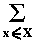(aj(x) - bj(x)) (ai(x) - bi(x)) .

When i = j, this equation defines the error variance within a given data layer.

Observation. The preceding equation facilitates calculation of the error variance of a composite map a(Fn)X as a function of a prespecified arithmetic operator applied during map overlay.

Example 1. When n layers are added, the composite error variance is given by [Ver94]:

sc =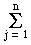sij .

Remark. Although error variance is always positive per layer, the error covariance may be positive or negative. Negative error covariance can imply that the error variance of the composite map may be lower than the variances of the indiviudal map layers. This would appear to contradict the absolute error assumption, namely, that absolute error of a map cannot be less than the error of its most erroneous component map. However, this appearance is false, since we are dealing with signed error, not the absolute value of an error measure.

Example 2. When a subtraction operator is applied to n layers, the composite error variance is given by [Ver94]:

sc =sij - 4 ·s1j .

Implementational Issues. The preceding method depends upon one's ability to measure variance and covariance for a given dataset. This implies that a and b coincide spatially (i.e., are coregistered), which may not hold in practice for irregular polygons. Note that rasterization can achieve some spatial alignment at the pixel level, albeit at the cost of additional spatial and attribute error.

#### 4.1.3.3. Case Study: Errors in Categorical Data.

Attributes that are classified taxonomically (i.e., categorized) may be difficult to map to an index set in a rigorous manner. Hence, one may want to deal with misclassification and erroneous assignment in a probabilistic fashion.

Concept. Errors in GIS data layers can be modelled via a contingency table approach.

Algorithm. Given attribute (e.g., cover) classes,

Step 1. Crosstabulate actual and estimated cover classes for a selected cell sample.
Step 2. Calculate the fraction of cells correctly classified for each layer.
Step 3. Determine map accuracy using inferential statistics, per the following discussion.

Remark. A minor modification of this algorithm uses the same number of samples from each cover class, to avoid error due to under-representation by small sample size.

Example. Consider employment of the logical and operator to match attributes between GIS data layers. Given the i-th layer with fraction Pr[Ei] of cells correctly classified, the composite map accuracy is given by:

Pr[Ec] = Pr[E1 and E2] = Pr[E1] · Pr[E2 | E1] ,

where the conditional probability Pr[E2 | E1] denotes the fraction of cells correctly classified in Layer 1 that are correctly classified in Layer 2. For more than two layers, the composite map accuracy is given by:

Pr[Ec] = Pr[E1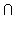E2...En] = Pr[E1] · Pr[E2 | E1] ·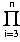Pr[E1 |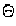(Ei)] ,

where(Ei) =Ej .

From these equations, minimum and maximum composite map accuracy can be computed as:

Pr[Ecmax] = min(Pr[Ei]), i = 1..N

Pr[Ecmin] = max(0, 1 -Pr[Ei]) ,

where Pr[Ei] denotes the fraction of cells in layer i that are misclassified.

Observation. The preceding results yield several concepts and observations concerning map accuracy and the logical and or set-intersection operator, as follows:

1. Composite map accuracy will at best equal the accuracy of the least accurate layer. This occurs when misclassified cells in each layer are coregistered with those in the least accurate layer.
2. At worst, composite map accuracy will be given by 1 -Pr[Ei] .
3. Accuracy as a function of n can be represented by a negative exponential curve.

The following related discussion of categorical coverages is taken from [Chr94].

Recall. Coregistration and co-location in map overlay is a fundamental operation upon which more complex GIS procedures are constructed.

Observation. Map overlay employs positional information to construct new polygons that share characteristics of the separate source layers or primary maps. One can think of overlay as visually implementing Venn diagrams applied to geographic database queries. Figure 4.1.3 is illustrative of the inheritance problems that result from misregistered data.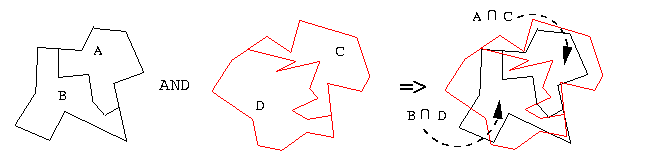Figure 4.1.3. Map overlay can be thought of as implementing Venn diagrams. Regions AC and BD inherit, or share characteristics of, their components.

Definition. A categorical coverage is a type of GIS map whose error model has spatial units that are adjusted on a continuous space (i.e., a subset of Rn) to reflect categorical distinctions.

Example. Categorical coverage is portrayed in Figure 4.1.4, which shows a three-class soil labelling problem. Assume that the labels are based on soil density (e.g., density(silt) > density(loam) > density(sand)). Since density measures can be expressed in terms of a subset of R, and the density-based labelling problem exemplified in Figure 4.1.4 occurs on the continuous domain R2, this is a categorical coverage problem.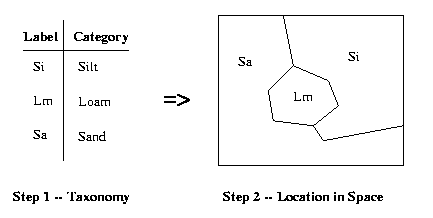Figure 4.1.4. Example of categorical coverage problem,
a type of map distinct from collection units.

Remark. In distinguishing categorical coverages from more usual collection maps, the important consideration is whether spatial attribute data take precedence.

Observations. The following instances are illustrative of some differences between categorical coverage and collection zone maps:

1. The term chloropleth maps previously referred to geographic maps that had categorized attributes added later -- the places or locations in space existed, then attributes were added. Presently, chloropleth refers to categorical maps derived from classed continuous distributions (e.g., the density example of Figure 4.1.4).

2. In collection zones, spatial boundaries are usually arbitrarily placed. In the past, autocorrelation was used to reduce boundary artifacts (e.g., measurement errors). Unfortunately, one must asume that some underlying classification has been obscured by zone boundary errors. Error correction involves a weight matrix that depicts relationships between collection units.

Example. In sociological studies, the geographic unit may be a city block or township, when in fact a person, firm, or household would be more appropriate. This difference can be due in part to the availability of statistical data over prespecified spatial domains (e.g., census data organized geographically).

3. In agronomic maps (e.g., soil surveys), similarlity or consistency of attribute type often indicates that the map was preceded by a taxonomic analysis. Positional accuracy, scale, and cartographic license become more important than in the collection zone case.

As part of this case study of errors in categorical data, we present the following high-level discussion of the effects of category error and overlay error in GIS maps.

Observation. The most common form of map overlay error is called a sliver, as shown in Figure 4.1.5. Some GIS become clogged with polygon representations of slivers and hence require spatial filtration. An example of this is the `MERGE` command in the IDRIS system.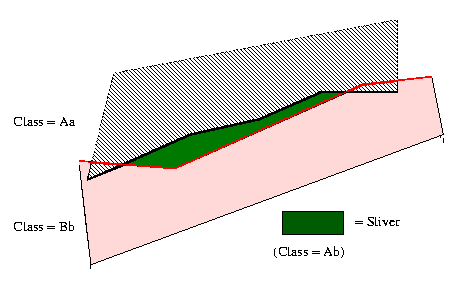Figure 4.1.5. "Slivers", the most common map overlay error.

Remark. While slivers are caused by positional error, the result is one or more classification errors (e.g., Ab in Figure 4.1.5). If the classes Aa and Bb are similar taxonomically, then Ab can be resolved to Aa, assuming that A is the dominant feature or attribute. In practice, this apparently obvious distinction can become obscured due to the interdependence of positional and classification errors.

Method. Existing techniques for analysis of categorical data can be adapted to GIS, for example:

• General linear model [Bis75], [Bib77]
• Log-Linear model: linear in logarithms of frequency-of-occurrence
• Logit/Probit model: linear in logarithms of ratios

Observation. The log-linear model allows a contingency table to be decomposed into effects in terms of a hierarchical model. Salient features of the log-linear model are listed as follows:

• The model is linear in logarithms because probabilities of independent events combine multiplicatively, per Bayes' Law.

• If an analysis of variance (ANOVA) is applied, then the following statements hold:

1. The terms of the ANOVA include an error term for each case or observation.
2. The log-linear model only considers cells of the contingency table (a good choice for categorical coverages, due to lack of an independent case).
3. Each table cell represents a Venn diagram combination due to the map overlay process.
This concludes our brief discussion of category-based errors. We next consider the error prediction and modelling process, which includes sensitivity analysis.

### 4.2. Prediction of Error and Complexity in GIS Algorithms

In order to employ statistical methods in analysis and prediction of GIS algorithm error, confidence limits are required for each dataset. Such measures can be propagated through error models, which can be designed to test algorithm sensitivity to a variety of input perturbations. In Section 4.2.1, we discuss the generation and interpretation of suitability maps, which can portray the utility of a dataset in a given GIS processing scenario. Section 4.2.2 overviews the process of GIS sensitivity analysis, and Sections 4.2.3 and 4.2.4 discuss the use of specialized software to determine error bounds on GIS algorithms. The analysis of complexity in GIS algorithms is summarized in Section 4.2.5.

#### 4.2.1. Suitability Map Generation

The following high-level model describes the process of suitability map generation from primary maps that describe observed physical reality (e.g., ground truth).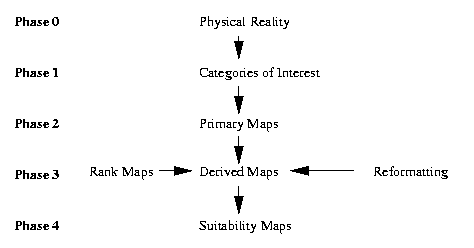Figure 4.2.1. Underlying model of suitability analysis applied to confidence limits.

Definition. A primary map is a map of an existing geographic entity of interest (e.g., geologic or vegetation map).

Definition. A rank map has attributes that are ordinal-, interval-, or ratio-valued and pertain to rankings that represent mathematical relations between attributes of more than one map. For example, a soil map could be ranked to determine potential for landslides or rate of water percolation.

Definition. Geographic suitability analysis applies a given operation or transformation to the attributes of one or more maps, for example, a rank map or an overlay.

Notation. Given n primary maps that are overlaid, let salient variables be defined as follows:

• P(n) denotes the number of polygons generated via intersection overlay of the n maps;

• ap,i, a scalar variable, denotes the attribute from map 1in in the p-th polygon of an intersection map; and

• wi, where 1in, denotes a scalar weight assigned to the i-th primary map.

Definition. The result of a suitability analysis restricted to the p-th polygon of the resultant suitability map has an attribute rp that is a function of the weight vector w = (w1, w2, ... , wn) and the attribute vector ap = (ap1, ap2, ... ,apn)`'`, as follows:

rp = f(w,ap) ,

which yields the resultant vector r = (r1, r2, ... , rP(n)).

Observation. If ap is replaced by point or line attributes (e.g., raster data is employed), then the preceding formulation attains full generality, including most commonly known GIS suitability analyses. In particular, the weighted intersection overlay is represented by:

rp = f(w,ap) =wi · ap,i , p = 1,2,...,P(n) .

Observation. The multidimensional scaling approach derives rp from the primary map attributes and a given attribute ci, where i = 1..n, as follows:

rp = f(w,ap) = (wi · ap,i)1/2 , p = 1,2,...,P(n) .

Note that the scalar ci is chosen for each of the primary or rank maps. Hence, c = (c1, c2, ..., cn).

Given the preceding theory, we now address the problem of sensitivity analysis of GIS algorithms.

#### 4.2.2. GIS Sensitivity Analysis

The difference between error propagation analysis (or error analysis) and sensitivity analysis is as follows:
• Geographic sensitivity analysis perturbs the primary maps to yield an output that is compared with the original map obtained by combining unperturbed inputs in the same was as the perturbed inputs were combined.

• Error propagation analysis uses error propagation theory to analyze a GIS algorithm's effect on the output map, and may also employ the aforemnetioned perturbation methods.

• In terms of the theory given in Section 4.2.1, sensitivity analysis is more interested in the operation f and on the effects upon f's output of perturbations in w and ap.

Definition. Confidence limits for attribute errors indicate the range of validity in an output attribute value given the error ranges in the input attribute values (e.g., in the primary maps).

Algorithm. The process of suitability map generation involves the following steps:

Step 1. Given m primary maps, create n rank maps.

Note: If m < n, then more than one unique rank map must be generated for a collection of primary maps. If m > n, then more than one primary map yields one rank map. Hence, we assume m = n, for convenience.

Step 2. Intersect the polygons of the n rank maps to yield the P(n) polygons of the suitability map. Attribute values are given by Equations (II) or (III).

Step 3. Transform primary map attributes to rank map attributes (interval or ratiometric data that connote a ranking), as follows:

Ti(bp,i) = ap,i , where i = 1..n, p = 1..P(n) .

Step 4.Transform rank map attributes to suitability map attributes via Equation (II) or (III).

Observation. One can represent the attributes of the suitability map as

rp =wi · ap,i =wi · Ti(bp,i) , p = 1,2,...,P(n) .

to obtain overlay suitability and

rp = (wi · (ap,i - ci)2 )1/2 = (wi · (Ti(bp,i) - ci)2 )1/2, p = 1,2,...,P(n) ,

for target suitability, where ci denotes the most or least preferred value of the i-th rank map.

The matrix product provides a concise, convenient expression for the preceding two equations. For example, consider the following equation:Likewise, given the ideal attributes I1 through In, if t = (r12, r22, ... , rP(n)2), then the target attributes are given by:We next consider measures of geographic sensitivity. Given an expression for Tn, the sensitivity of individual polygons can be determined. At least five sensitivity measures for entire maps have been defined by Lodwick [Lod94], as follows:

1. Attribute Sensitivity Measures (ASMs) describe the net magnitude of changes in attribute values from their unperturbed values.

2. Position Sensitivity Measures (PSMs) describe how many of the attributes change with respect to their rank order from their unperturbed (original or source) ranking.

3. Map Removal Sensitivity Measures (MRSMs) describe how the sensitivity associated with removing a set of maps from a suitability analysis. This measure derives from perturbation of the weight vector w that is equal in magnitude byt opposite in sign to its value in the unperturbed suitability analysis.

4. Polygon Sensitivity Measures (PoSMs) determine which polygons are more sensitive to perturbations.

5. Areal Sensitivity Measures (ArSMs) compute the total area over which attribute changes occur.

We examine each type of sensitivity measure, as follows.

1. Attribute Sensitivity Measures (ASMs):

Let rp denote the attirbute resulting from an absence of perturbation, and let rp result from nonzero perturbation. When sensitivity analysis does not require removal of one or more maps from the suitability analysis, the weighted ASM is given by:

m1(s,r,r) =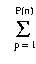sp · | rp - rp | ,

where the weight vector s = (s1, s2, ... , sP(n)).

Notes:

· If sp = 1, then m1 is said to be unweighted.

· If sp = Ap, where Ap denotes the area of the p-th polygon, then m1 is said to be an areal-weighted sensitivity measure.

· A normalized areal-weighted attribute sensitivity measure can be computed by setting the p-th weight, as follows:

sp = Ap /Ap .

2. Position Sensitivity Measures (PSMs):
Let F denote the union of the attributes of n rank maps denoted by rp 1pP(n). Let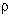: F -> N denote the operation that assigns rank order (e.g., from low to high) to all the attributes of the P(n) polygons in the n overlaid suitability maps. The perturbed attributes are denoted by rp.

The weighted position sensitivity measure (e.g.,weighted for position in a given rank ordering scheme) is given by:

m2(s,r,r) =sp · |(rp) -(rp) | ,

where the weight vector s = (s1, s2, ... , sP(n)) was defined previously.

Notes:

· If sp = 1,then m2 is said to be unweighted.

· If sp = Ap, then m2 is termed an areal-weighted rank sensitivity measure.

· If sp portrays the proportion of total map area occupied by the p-th polygon (per the preceding section on ASMs), then m2 is said to be a normalized areal-weighted rank sensitivity measure.

3. Map Removal Sensitivity Measures (MRSMs):
Removing one or more maps from a suitability analysis tends to lower the perturbed attribute values rp for all polygons, due to variance reduction. This removal represents a type of analytical bias that can be compensated by dividing each suitability map attribute by the number of primary maps n.

For example, if k denotes the number of primary maps remaining after map removal, then the corresponding MRSM is given by:

m3(s,r,r) =sp · | (rp / n) - (rp / k) | ,

where the weight vector s was defined previously.

4. Polygon Sensitivity Measures (PoSMs):

To find which attributes undergo maximum change as a result of perturbing the primary maps, one must find the polygon index or indices for which | rp - rp | is maximized.

The polygons that undergo maximum change have indices in the set

J = { (jN : | rj - rj | =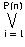| ri - ri | } .

The polygon sensitivity measure is thus given by

m4(s,r,r) =| ri - ri | .

5. Area Sensitivity Measures (ArSMs):
The area that undergoes a change in attribute values given perturbation of the primary maps is described by the following area sensitivity measure:

m5(s,r,r) =sp · Ap ,

where sp = 1 if rp = rp or sp = 0 otherwise.

Note: If sp = | rp - rp |, then we obtain a relative area sensitivity measure.

Algorithm. The following procedure outlines the methodology employed in computing geographic sensitivity analyses. Given a GIS that can a) perform intersection overlay and b) access individual attributes of n primary maps, perform the following steps:

Step 1. Obtain rp from perturbed inputs by using r = T · w or t = T · w, as given previously.

Step 2. Measure sensitivity using m1 through m5, as discussed in the preceding sections.

Step 3. If the probability distribution associated with Monte Carlo simulation is known, then Monte Carlo simulation of r = T · w or t = T · w would produce resultant attributes that could be analyzed statistically.

#### 4.2.3. Confidence Limits Associated with Geographic Sensitivity.

LEFT OFF HERE (middle of p.28 in notes)

#### 4.2.5. Overview of Complexity Analysis for GIS Algorithms### References.

[Bib77] Bibby, J. "The general linear model: A cautionary tale", in Analysis of Survey Data 2:35-80, Eds. C. O'Muircheartaigh and C. Payne, New York: John Wiley (1977).

[Bis75] Bishop, Y., S. Fienberg, and P. Holland. Discrete Multivariate Analysis: Theory and Practice, Boston, MA: MIT Press (1975).

[Chr94] Chrisman, N.R. "Modelling error in overlaid categorical maps", in Accuracy of Spatial Databases, Eds. M. Goodchild and S. Gopal, London: Taylor and Francis, Second Printing (1994).

[Goo78] Goodchild, M.F. "Statistical aspects of the polygon overlay problem", Harvard Papers on Geographic Information Systems, Volume 6, Reading, MA: Addison-Wesley (1978).

[McA71] McAlpine, J.R. and B.G. Cook. "Data reliability from map overlay", in Proceedings of the 43rd Congress of the Australian and New Zealand Association for the Advancement of Science (1971).

[Ver94] Veregin, H. "Error modeling for the map overlay operation", in Accuracy of Spatial Databases, Eds. M. Goodchild and S. Gopal, London: Taylor and Francis, Second Printing (1994).

This concludes our introductory discussion of GIS issues.
We next consider computational problems related to GIS features.